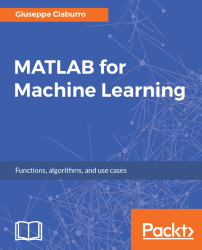•#### MATLAB for Machine Learning#### Overview of this book

MATLAB is the language of choice for many researchers and mathematics experts for machine learning. This book will help you build a foundation in machine learning using MATLAB for beginners. You’ll start by getting your system ready with t he MATLAB environment for machine learning and you’ll see how to easily interact with the Matlab workspace. We’ll then move on to data cleansing, mining and analyzing various data types in machine learning and you’ll see how to display data values on a plot. Next, you’ll get to know about the different types of regression techniques and how to apply them to your data using the MATLAB functions. You’ll understand the basic concepts of neural networks and perform data fitting, pattern recognition, and clustering analysis. Finally, you’ll explore feature selection and extraction techniques for dimensionality reduction for performance improvement. At the end of the book, you will learn to put it all together into real-world cases covering major machine learning algorithms and be comfortable in performing machine learning with MATLAB.
Title PageCreditsForewordwww.PacktPub.comCustomer FeedbackPrefaceFree Chapter
Getting Started with MATLAB Machine LearningImporting and Organizing Data in MATLABFrom Data to Knowledge DiscoveryFinding Relationships between Variables - Regression TechniquesPattern Recognition through Classification AlgorithmsIdentifying Groups of Data Using Clustering MethodsSimulation of Human Thinking - Artificial Neural NetworksImproving the Performance of the Machine Learning Model - Dimensionality ReductionMachine Learning in Practice## Introduction to clustering

In clustering, as in classification, we are interested in finding the law that allows us to assign observations to the correct class. But unlike classification, we also have to find a plausible subdivision of our classes.

While in classification, we have some help from the target (the classification provided in the training set), in the case of clustering, we cannot rely on any additional information and we have to deduce the classes by studying spatial distribution of data.

The areas where data is thickened corresponds to similar observation groups. If we can identify observations that are similar to each other and at the same time different from those of another cluster, we can assume that these two clusters match different conditions. At this point, there are two things we need to go more deeply into:

• How to measure similarity
• How to define a grouping

The concept of distance and how to define a group are the two ingredients that describe a clustering algorithm.